# topic diversity介绍

Joseph A. Konstan教授(coursera.明大.推荐系统课程)在2005年《Improving Recommendation Lists Through Topic Diversification》中提出了主题多样性。虽然这篇paper比较老，但比较经典，值得一看：

# 1.介绍

## 1.1 贡献

• 主题多样性(topic diversification)：我们提出了一种方法，根据该活跃用户的完整范围的兴趣，来平衡top-N推荐列表。我们的新方法会同时考虑：suggestions给出的accuracy，以及在特定主题上的用户兴趣范围。主题多样性的分析包含：user-based CF和item-based CF.
• Intra-list相似度指标（similarity metric）：
• accuracy vs. satisfaction：

# 3.评估指标

## 3.1 Accuracy Metrics

Accuracy metrics主要有两个：

## 3.2 Accuracy之外

### 3.2.2 Noverlty和Serendipity

Novelty和serendipity指标可以衡量推荐的”非显而易见性（non-obviousness）”，避免“随机选取（cherry picking）”。对于serendipity的一些简单measure，可以采用推荐items的平均流行度。分值越低表示越高的senrendipity。

## 3.3 Intra-List Similarity

$ILS(P_{w_i}) = \frac{\sum\limits_{b_k \in \Im P_{w_i}} \sum\limits_{b_e \in \Im P_{w_i}, b_k \neq b_e} c_o(b_k, b_e)}{2}$

…(5)

$\forall \delta_i, \delta_j \in S_N: ILS(P_{w_i} \circ \delta_i) = ILS(P_{w_i} \circ \delta_j)$

…(6)

# 4.topic diversification

acurracy指标的一个主要问题是，它不能捕获更宽泛的用户满意度，会隐藏在已存在系统中的一些明显缺陷。例如，推荐出一个非常相似items的列表（比如：对于一个很少使用的用户，根据author、genre、topic推出），尽管该列表的平均accuracy可能很高。

## 4.1 Taxonomy-based Similarity指标

classification taxonomies存在不同领域。Amazon.com为books/DVSs/CDs/电子产品等制作了非常大的taxonomies。图1表示一个sample taxonomy。另外，在Amazon.com上的所有商品的内容描述与它们的domain taxonomies相关。特征化的主题可以包含：author、genre、audience。## 4.2 topic diversification算法## 4.4 渗透压（Osmotic Pressure）类比

dissimilarity效应与分子生物学上的渗透压和选择性渗透（selective permeability）相类似。将商品$b_o$（它来自兴趣$d_o$的特定领域）稳定插入到推荐列表中，等价于：从来自一个特定物质的分子通过细胞膜传到细胞质中。随着浓度$d_o$（它属于膜的选择性通透性）的增加，来自其它物质d的分子b的压力会上升。对于一个给定主题$d_p$，当压力(pressure)足够高时，它最好的商品$b_p$可能“散开（diffuse）“到推荐列表中，尽管他们的原始排序（original rank）$P_{w_i}^{-1}(b)$可能不如来自流行域（prevailing domain）$d_o$。相应的，对于$d_p$的压力会下降，为另一个压力上升的domain铺路。

# 参考

• i) 所有这些都被experts认为是“major”搜索引擎s
• ii)大多数用户和metasearch engines更偏向于他们来执行它们的搜索## 3.1 处理individual rankings

$K(c)=\sum\limits_{i=1}^m (k+1 - r_i(c))$

…(2)

• m: 表示所使用的component engines的数目
• c: 表示在component list中的单个结果
• k: 在每个component list中的items数目
• $r_i(c)$是该item被分配给第i个component engine得到的ranking• 1.$c_2$在更多的input rankings中出现，相应的，根据民主对称性(democratic symmetry) Saari(2000)
• 2.$c_2$在超过一半的input rankings中出现，因此根据孔多塞标准（Condorcet criterion），它是一个spam entry的概率很低

$R(c)=m log(n_c K(c))$

…(3)

# 1.介绍# 3.方法

## 3.1 低维商品embeddings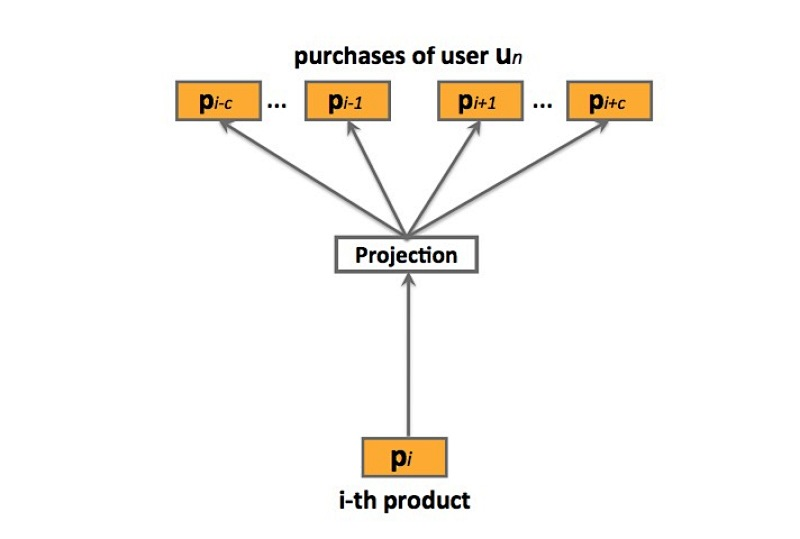prod2vec：prod2vec模型会将一个购买序列看成是一个“句子”，在这个序列中的商品看成是“词”。详见图2, 更特殊的，prod2vec使用skip-gram模型来学习商品表示，通过以下的最大化目标函数：

$L = \sum_{s \in S} \sum_{p_i \in s} \sum_{-c \leq j \leq c, j \neq 0} log p(p_{i+j} | p_i)$

…(3.1)

$P(p_{i+j}| p_i) = \frac{exp({v_{p_i}^T v_{p_{i+j}}'})} {\sum_{p=1}^P exp{(v_{p_i}^T v_p')}}$

…(3.2)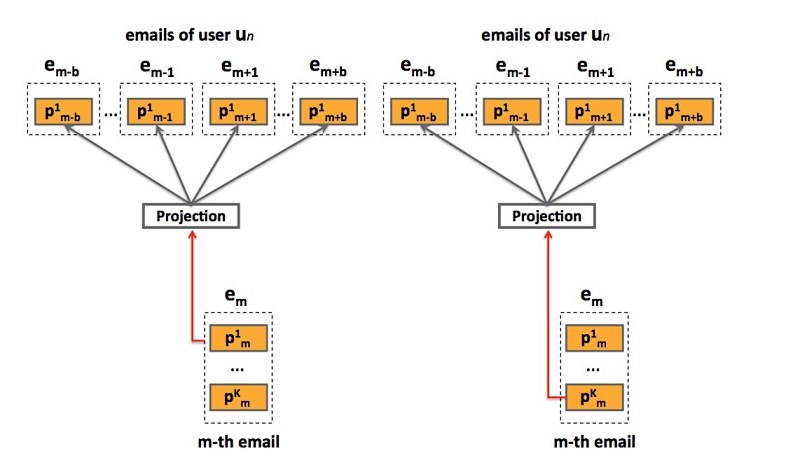bagged-prod2vec：为了对多个商品同时购买的行为做出解释，我们提出了一个修改版本的skip-gram模型，它引入了一个概念：购物袋（shopping bag）。如图3所示，该模型会在email receipts级别进行操作，而非在商品级别。通过对email序列s上的一个修改版目标函数进行最大化，来学习商品向量表示：

$L = \sum_{s \in S} \sum_{e_m \in s} \sum_{-n \leq j \leq n, j\neq 0} \sum_{k=1,...,T_m} log P(e_{m+j}| p_{mk})$

…(3.3)

$P(e_{m+j} \mid p_{mk}) = P(p_{m+j,1} \mid p_{mk} ) \times ... \times P(p_{m+j,T_m} \mid p_{mk})$

learning：该模型使用SGA（ stochastic gradient ascent）进行最优化，很适合大规模问题。然而，在(3.1)和(3.3)中的梯度计算$\Delta L$，很适合词汇size P，实际任务中，计算开销会随着P的增大而变得很昂贵，很容易达到上百万的商品。另一方面，我们使用negative sampling方法，它能显著减小计算复杂度。

## 3.2 prod-2-prod预测模型

prod2vec-topK：给定一个购买商品，该方法会为所有在词汇表中的商品计算cosine相似度，并推荐最相似商品的top K

prod2vec-cluster：为了能做出更多样化(diverse)的推荐，我们考虑将相似商品分组成聚类，从与之前购买商品相关的聚类中推荐商品。我们应用K-means聚类算法来在hadoop FS上实现，将商品基于cosine相似度进行聚类。我们假设：在从聚类$c_i$上进行一个购买后、再从任意第C个聚类中进行一次购买的行为，符合一个多项式分布（multinomial distribution）$M_u(\theta_{i1}, \theta_{i2}, ..., \theta_{iC})$，其中$\theta_{ij}$是从聚类$c_i$中进行一次购买后、接着从聚类$c_j$中进行一次购买的概率。为了估计参数$\theta_{ij}$，对于每个i和j，我们采用一个最大似然方法：

$\hat {\theta_{ij}} = \frac{c_i购买后跟c_j的次数}{c_i购买的总数}$ …(3.4)

• count of ci purchases: c_i购买的数目
• # of times ci purchase was followed by cj: c_i购买后跟c_j的次数

## 3.3 User-to-product预测模型

user2vec：受paragraph2vec算法的启发，user2vec模型会同时学习商品和用户的特征表示，它会将用户当成是一个“全局上下文”。这样的模型如图4所示。训练数据集来自于用户购买序列S，它会包含$u_n$和其它已购商品（通过购买时间序排列），$u_n = (p_{n1}, p_{n2}, ..., p_{nU_n})$，其中$U_n$表示用户$u_n$购买的items数目。在训练期间，用户向量会被更新，来预测从他的email receipts中的商品，其中，学到的product vectors会预测在上下文中的其它商品。出于表示的简洁性，在下面，我们会表示no-bagged版本的模型，注意，使用bagged版本进行扩展也很方便。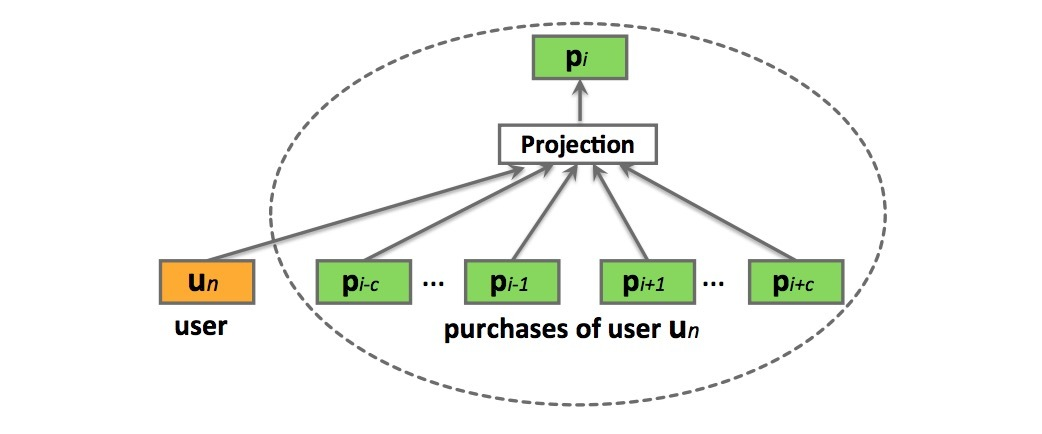$L = \sum_{s \in S} (\sum_{u_n \in s} log P(u_n | p_{n1}: p_{nU_n}) + \sum_{p_{n_i} \in u_n} log P(p_{ni}| p_{n,i-c}: p_{n,i+c,u_n}))$

…(3.5)

$P(p_{ni}|p_{n,i-c}: p_{n,i+c}, u_n) = \frac{e^{\bar{v}^T v_{p_{ni}}'}}{ \sum_{p=1}^{V} e^{\bar{v}^T v_p'}}$ …(3.6)

$\bar{v} = \frac{1}{2c+1} (v_{u_n} + \sum_{-c \leq j \leq c ,j \neq 0} v_{p_{n,i+j}})$ …(3.7)

$P(u_n | p_{n1}: p_{nU_n}) = \frac{e^{\bar{v}_n^T v_{u_n}'}} {\sum_{p=1}^V e^{\bar{v}_n^T v_p'}}$ …(3.8)

$\bar{v}_n = \frac{1}{U_n} \sum_{i=1}^{U_n} v_{p_{ni}}$

…(3.9)

user2vec模型的一个主要优点是，商品推荐是基于该用户的购买历史进行量身定制的。然而，缺点是，该需要需要非常频繁地进行更新，不同于product-to-product方法，它可以长期是相关的，而user-to-product推荐需要经常变化来对最近购买行为做出解释。

# 4.实验及其它

## 4.4 推荐预测商品

• 1) prod2vec-topK：使用数据集$D_p$进行训练，其中，商品向量通过对购买序列s通过极大似然估计进行学习。给定一个商品$p_i$，通过向量空间计算cosine相似度选择topK个相似商品。
• 2) bagged-prod2vec-topK：使用$D_p$进行训练，其中商品向量通过email序列s通过极大似然估计进行学习。对于给定商品$p_i$，通过选择在结合向量空间计算cosine相似度选择topK个相似商品。
• 3) bagged-prod2vec-cluster: 与bagged-prod2vec模型的训练类似，接着将商品向量聚类成C个聚类，并计算它们间的转移概率。接着标识出$p_i$属于哪个聚类（例如：$p_i \in c_i$），我们根据$c_i$的转移概率对各聚类进行排序，取出top个聚类，然后聚出这些top聚类的商品通过计算与$p_i$的cosine相似度进行排序，其中每个聚类的top $K_c$被用于推荐（$\sum {K_c} = K$）。 bagged-prod2vec-cluster与 bagged-prod2vec的预测结果如表2所示。可以看到聚类方法多样性更好。
• 4) user2vec：使用$D_p$进行训练，其中商品向量和用户向量通过极大似然估计进行学习。给定一个用户$u_n$，通过计算$u_n$用户向量与所有商品向量的cosine相似度，检索出top K近邻商品。
• 5) co-purchase：对于每个购买pair：$(p_i, p_j)$，计算频率$F_{(p_i,p_j)}$，其中$i=1,...,P, j=1,...,P$，商品$p_j$在商品$p_i$之后立即购买。接着，给商品$p_i$的推荐通过频率$F_{(p_i,p_j)}, j=1,...,P$进行排序，取topK商品。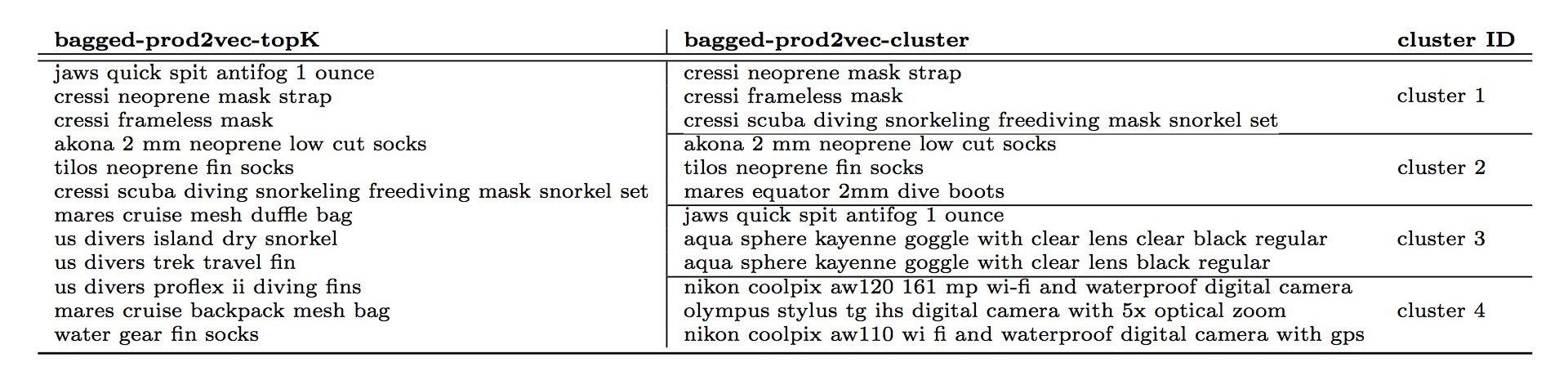$d_j = sim_j \cdot \alpha^{t_d - t_i}$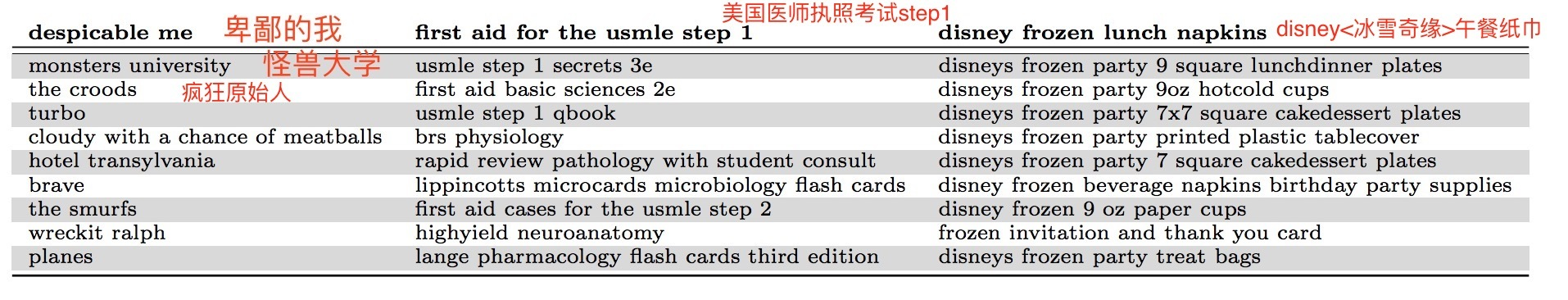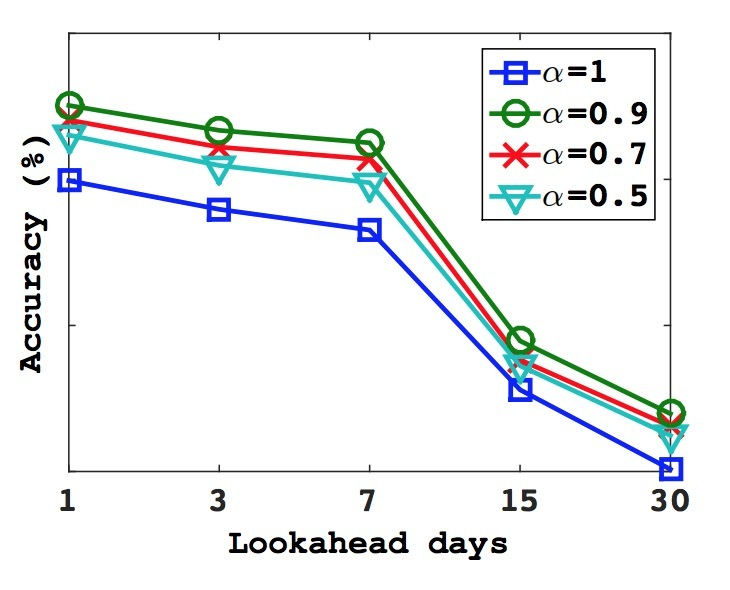# 1.介绍

## 2.相关工作

NLP的字符级特征（Character level features）：与我们工作相关的另一个研究领域是，NLP的字符级模型，它直接从字符序列中学习表示。这种模型的最高级方法是RNN，应用到语言建模（Mikolov 2012）、文本归一化（Chrupala, 2014）,、词性标注(Ling.2015)、parsing（Ballesterors.2015）。该模型的另一个家族是在字符上训练的CNN，它可以被应用到：词性标注（Santos,2014）、情感分析（dos Santos.2014）、文本分类（zhang.2015）、语言建模（Kim.2016）。(Sperr.2013)提出了基于受限波尔茨曼机的语言模型，其中词被编码成字符级别n-grams的一个集合。最后，在机器翻译中的最近工作也提出了使用subword units来获取罕见词的表示。（Sennrich.2016）

# 3.模型

## 1.1 通用模型

$\sum_{t=1}^{T}\sum_{c \in {C_t}}log p(w_c|w_t)$

$p(w_c|w_t)=\frac{e^{s(w_t,w_c)}}{\sum_{j=1}^{W}e^{s(w_t,j)}}$

$log(1+e^{-s(w_t,w_c)})+\sum_{n \in {N_{t,c}}} log(1+e^{s(w_t,n)})$

$\sum_{t=1}^{T}\sum_{c \in C_t}l(s(w_t,w_c))+\sum_{n \in N_{t,c}}l(-s(w_t,n))$

$w_t$和上下文词$w_c$采用标量积：$s(w_t,w_c)=u_{w_t}^{T}v_{w_c}$

## 1.2 Subword模型

$s(w,c)=\sum_{g \in {G_w}}z_g^Tv_c$

# 1.4 试验

• 小数据集：100维, 中数据集：200维，完整数据集:300维.
• skip-gram baseline learning-rate: 0.025; CBOW: 0.05, 新模型:0.05

• 英文：使用 WS353 (Finkelstein et al.2001)以及 RW (Luong et al.2013)
• 德文：Gur65, Gur350,ZG222(Gurevych, 2005; Zesch and Gurevych, 2006）
• 法文：RG65(Joubarne and Inkpen, 2011)
• 西班牙文：WS353(Hassan and Mihalcea, 2009)# 2.高效文本分类tricks

Mikolov等在中提到了多种高效文本分类的tricks，并提出了fastText。它的分类速度快，又不失精准。在标准多核CPU上训练，超过10亿词上只需要10分钟左右；而对50w的句子，在312K个分类上进行分类，1分钟之内即可完成。听上去有些小激动。

## 2.1 模型架构

baseline: 对于句子分类，简单又有效的baseline为：BOW向量 + 一个线性分类器(LR或SVM)。$-\frac{1}{N}\sum_{n=1}^{N}y_nlog(f(BAx_n))$

### Hierarchical softmax

$P(n_{l+1})=\prod_{i=1}^{l}P(n_i)$

### N-gram features

BOW与词序无关，显式采用该顺序的计算开销通常很大。作为替代，我们使用bag-of-n-grams作为额外的特征来捕获一些关于局部词序的部分信息(partial information)。这在惯例上很有效（Wang and Manning, 2012).

## 2.2 实验评测

fastText在两个不同的任务上进行评测。首先，会比较在情感分析（sentiment analysis）问题上的文本分类器。模型的实现可以使用Vowpal Wabbit library，但实际上使用的定制版本要比它快2-5x倍。

### 情感分析(Sentiment analysis)### 标签预测# 介绍

• X是一个任意输入空间
• $A=\lbrace 1, \cdots, k \rbrace$是一个actions集合

• 1.抽取$(x, \vec{r}) \sim D$，并公布x
• 2.算法会选择一个action $a \in A$，可以是一个关于x和历史信息的函数
• 3.公布action a的reward为$r_a$，但不会公布$a'\neq a$是$r_{a'}$

$V^h := \underset{(x,r) \sim D}{E} [r_{h(x)}]$

• 1.监督学习：我们可以学到一个regressor $s: X \times A \rightarrow [0,1]$，它可以在观察到的events上基于action a和其它信息x，训练来预测reward。从该regressor，一个policy可以根据$h(x)=argmax_{a \in A} s(x,a)$来生成。该方法的缺点是，argmax会超出在训练数据中未包含的选择集合，因此不能被泛化。这可以通过一些极端情况进行验证。假设，有两个actions a和b，其中action a出现$10^6$次，action b出现$10^2$次。由于action b的次数比例只占$10^{-4}$，一个学习算法会强迫在预测$r_a$和$r_b$的期望值间做权衡，并压倒性地偏向于估计$r_a$，代价是精准估计$r_b$。然而，在应用上，action b可能会被argmax选中。当action b出现0次时，该问题会更糟糕
• 2.Bandit方法：略
• 3.Contextual Bandits：略
• 4.Exploration Scavenging：略

• 1.对于每个event $(x,a,r_a)$，使用回归(regression)来估计logging policy会选择action a的概率(probability) 为$\hat{\pi}(a \mid x)$。这里，“probability”是随时间变化的——我们可以想像：在不同时间点，采用一个均匀随机的方式从policies集合（可能是deterministic）中抽取。
• 2.对于每个event $(x,a,r_a)$，根据$(x,a,r_a, \frac{1}{max \lbrace \hat{\pi}(a \mid x), \tau \rbrace})$，创建一个synthetic controlled contextual bandit event，其中$\tau > 0$是一些参数。$\frac{1} { max \lbrace \hat{\pi}(a \mid x), \tau \rbrace}$这个量是一个importance weight，它指定了当前event对于训练是有多重要。需要明确的是，参数$\tau$对于数值稳定性很重要。
• 3.应用一个offline contextual bandit算法到synthetic contextual bandit events集合上。在我们第二个实验结果的集合（4.2节）中，采用的argmax regressor的变种使用了两个关键修改之处：(a) 我们将argmax的范围限定在具有正概率的那些actions上 (b) 我们对events进行importance weight，以便训练过程会强调对于每个action平等的好估计。需要强调的是，在本paper中的理论分析可以应用于对于在contextual bandit events学习上的任何算法——我们选择该方法是因为它很容易在已存在方法上进行简单修改。

• 1.在当给定特征x时，logging policy会确定式(deterministically)选中一个action (ad) a，$\hat{\pi}(a \mid x)$意味着什么，？基本observation：一个policy如果在day 1会确定式选中action a、接着在day 2会确定选中action b，这可以被看成是：(当events数目在每天都相同时，并且events间是IID的），在action a和b间使用概率0.5进行随机化。因而，在logged events的时间跨度上，$\hat{\pi}(a \mid x)$是一个给定特征x关于action a被展示的期望频率的估计。第3节中，我们会展示该方法在期望上是合理的，它提供了关于new policy的值的一种无偏估计。
• 2.在$\hat{\pi}(a \mid x)$上的客观误差（inevitable errors）是如何影响该过程的？结果表明它们有影响，取决于$\tau$。对于非常小的$\tau$值，$\hat{\pi}(a \mid x)$的估计必须极其精准来生成好的效果；而对于更大的值，会需要更小的accuracy。第3.1节会证明健壮性
• 3.参数$\tau$是如何影响最终结果的？当在估计过程中创建一个bias时，结果表明该bias的形式是很轻微的并且相对合理的——基于条件x具有低频展示的actions具有一个under-estimated value。这与期望的对于没有频率的actions的限制是一致的。

# 2.问题设定和假设

• $\pi_1, \cdots, \pi_T$是T个policies，

• $(x,r)$按第1节所述的方式从D中抽取
• action $a \sim \pi_t(x)$根据第t个policy被选中。

offline policy estimator

$S = \lbrace (x_t, a_t, r_{t,a_t}) \rbrace_{t=1}^T$

…(1)

$\hat{V}_{\hat{\pi}}^h(S) = \frac{1}{|S|} \sum\limits_{(x,a,r) \in S} \frac{r_a I (h(x)=a)}{max \lbrace \hat{\pi}(a \mid x), \tau \rbrace}$

…(2)

# 3.理论成果

• 1.我们具有一个policy estimation阶段，其中我们估计未知的logging policy;
• 2.我们具有一个policy optimization阶段，其中我们会使用我们估计的logging policy。

logging policy $\pi_t$可能是确定的(deterministic)，这意味着：基于logging policy中的随机化的常用方法是不可用的。我们会在下面展示：当现实情况是IID条件、并且policy会随actions的不同而变化时，这是ok的。我们会有效使用算法中的随机化标准方法来替代现实中的随机

$\pi(a | x) = \underset{t \sim UNIF(1,\cdots,T)}{E} [\pi_t (a | x)]$

…(3)

stochastic policy $\pi$会在T policies $\pi_t$上随机均匀选择一个action。我们的第一个结果是，当现实中根据$\pi$或者policies序列$\pi_t$进行选择actions时，我们的estimator的期望值与是相同的。尽管该结果和它的证明是简单的，它是本paper后面部分的基础。注意，policies $\pi_t$可以是任意的，但我们会假设，它们不依赖于用于evaluation的数据。该假设只对证明来说是必要的，可以在实际中放宽些，如4.1节。

$E_{S \sim (D,\pi_i(\cdot|x))_{i=1}^T} \hat{V}_{\hat{\pi}}^h(S) = E_{(x,\vec{r}) \sim D, a \sim \pi(\cdot |x)} \frac{r_a I (h(x)=a)}{max \lbrace \hat{\pi}(a|x), \tau \rbrace}$

…(4)

S就是上面指的dataset。

## 3.1 Policy Estimation

$reg(x)= \underset{a \in A}{max} [(\pi(a \mid x) - \hat{\pi}(a \mid x))^2]$

…(5)

• $I(\cdot)$表示了indicator function
• $\pi(a \mid x)$是logging policy在输入x上选择action a的概率
• $\hat{V}_{\hat{\pi}}^h$是由等式(2)基于参数$\tau$的定义

• $\hat{\pi}$是从X到在actions A分布上的任何函数
• $h: X \rightarrow A$是任意的deterministic policy。
• $V^h(x) = E_{r \sim D(\cdot \mid x)}[r_{h(x)}]$表示在input x上执行policy h的期望值

$E_x [ I(\pi(h(x) | x) \geq \tau) \cdot (V^h(x) - \frac{\sqrt{reg(x)}}{\tau}) ] \leq E[\hat{V}_{\hat{\pi}}^h] \leq V^h + E_x [ I(\pi(h(x) \mid x) \geq \tau) \cdot \frac{\sqrt{reg(x)}}{\tau} ]$

$E_x [ I(\pi(h(x) | x) \geq \tau) \cdot V^h(x)] \leq E[\hat{V}_{\hat{\pi}}^h] \leq V^h$

$E[\hat{V}_{\hat{\pi}}^h]=\pi(a_1 \mid x) / \hat{\pi}(a_1 \mid x) = (\tau + \epsilon) / \tau$

$E[\hat{V}_{\hat{\pi}}^h] - V^h = |(\tau+\epsilon)/\tau - 1| = \epsilon /\tau$

$(\pi(a_1 \mid x) - \hat{\pi}(a_1 \mid x))^2 = \epsilon^2$

## 3.2 Policy Optimization

$\pi(a | x) = E_{t \sim UNIF(1,\cdots,T)}[\pi_t(a | x)]$

3.1节的结果可以应用到policy optimization问题上。然而，注意，该数据被看成是通过从一个关于T个policies $\pi_i, \cdots, \pi_T$的执行序列上抽取得到，而非从$\pi$上进行T个抽取得到。

• $\hat{\pi}$是任意从X到actions A分布的函数
• H是任意deterministic policies的集合

• $\tilde{H}=\lbrace h \in H \mid \pi(h(x) \mid x) > \tau, \forall x \in X \rbrace$
• $\tilde{h} = argmax_{h \in \tilde{H}} \lbrace V^h \rbrace$

• $\hat{h} = argmax_{h \in H} \lbrace \hat{V}_{\hat{\pi}}^h \rbrace$是最大化在等式(2)中定义的empirical value estimator的hypothesis。

$V^{\hat{h}} \geq V^{\tilde{h}} - \frac{2}{\tau} (\sqrt{E_x[reg(x)]} + \sqrt{\frac{ln(2|H|/\delta)}{2T}})$

…(6)

# 4.评估

## 4.1 实验I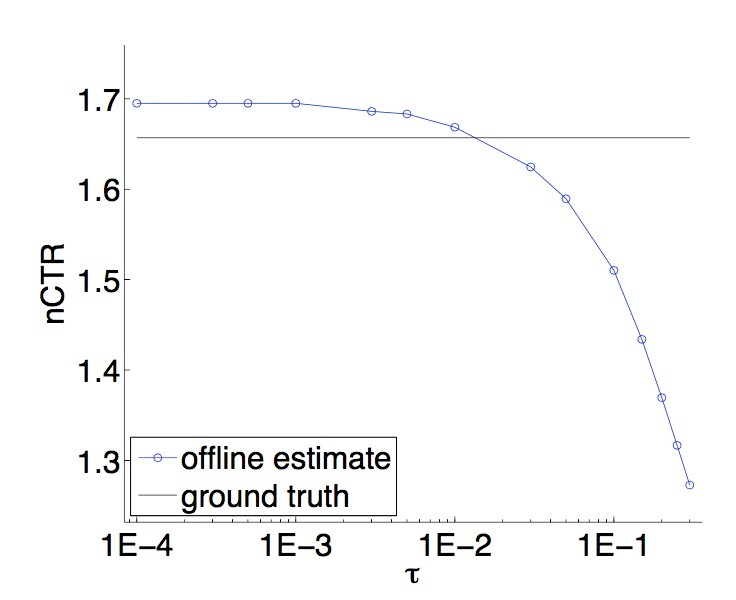## 4.2 实验II

$\hat{V}_{\hat{\pi}}^h = \frac{1}{T} \sum\limits_{t=1}^T \frac{y_t I(h(x_t)=a_t)}{max \lbrace \hat{\pi}(a_t | x_t) , \tau \rbrace}$

…(7)

Interval列的计算使用Chernoff bound相对熵的形式，$\delta=0.05$持有假设：变量是IID的，（即：在我们的case中，用于estimator计算的样本）。注意，该计算有些复杂，因为变量的范围是$[0, 1/\tau]$，而非常见的$[0, 1]$。这可以通过乘以$\tau$来进行缩放，应用到bound上，接着将结果乘以$1/\tau$进行缩放。

“random” policy指的是，从feasible ads集合中随机选择：$Random(x)=a \sim UNIF(C(X))$，其中$UNIF(\cdot)$表示均匀分布。

“Naive” policy对应于理论上有缺陷的监督学习方法（在介绍中详细说过）。这种policy的evaluation相当昂贵，需要每个example每个ad进行一个evaluation，在此，test set的size被减小到一个click 8373个examples，这会减小结果的重要性。通过按时间先后顺序选择在test set中的第一个events（例如：在training set中与它们最相似的events），我们会将该结果偏向于naive policy。然而，naive policy会收到0 reward，这要比其它方法都要小很多。使用这种evaluation的一个可能担忧之处是，naive policy总是会找到那些不会被explored的good ads。一种快速验证表明：这是不正确的——naive argmax会简单地做出难以置信的选择。注意，我们只上报了evaluation against $\tau=0.05$，因为evaluation against $\tau=0.01$并不明显，尽管reward仍是0.

“Learned” policies会依赖于$\tau$。如定理3.2所示，随着$\tau$是减小，我们相竞争的hypotheses的有效集合是增加的，从而允许learned policy具有更好的效果。确实，当我们把$\tau$从0.05减小到0.01时，learned policy和random policy的估计会同时提升。

empirical结果与理论方法大致是consistent的——它们提供了一个一致的关于policy value的最坏估计，不过它们具有足够动态的范围来区分learned polices vs. random policies，以及learned policies在更大空间(更小$\tau$)vs.更小空间(更大$\tau$)上，理论上不完善的naive方法 vs 合理的方法(在ads的explored空间上进行选择)。